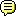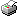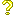Register (or login) on our website and you will not see this ad.doc_holiday(fountain of knowledge) Fri 12-Mar-21 12:29:35# Can you post some pings?

Hi AAISPers --

Would anyone be willing to post pings from their routers to AAISP's first hop? I am considering moving to AAISP, and this would be useful to understand at least from where you are in the country what you are getting.

Here's a current set of pings from my router through FTTP to the first hop into IDnet's core. No load from my router.

64 bytes from 212.69.63.77: icmp_seq=54 ttl=253 time=7.552 ms
64 bytes from 212.69.63.77: icmp_seq=55 ttl=253 time=7.752 ms
64 bytes from 212.69.63.77: icmp_seq=56 ttl=253 time=7.913 ms
64 bytes from 212.69.63.77: icmp_seq=57 ttl=253 time=7.815 ms
64 bytes from 212.69.63.77: icmp_seq=58 ttl=253 time=8.284 ms
64 bytes from 212.69.63.77: icmp_seq=59 ttl=253 time=10.137 ms
64 bytes from 212.69.63.77: icmp_seq=60 ttl=253 time=8.363 ms
64 bytes from 212.69.63.77: icmp_seq=61 ttl=253 time=40.957 ms
64 bytes from 212.69.63.77: icmp_seq=62 ttl=253 time=51.770 ms
64 bytes from 212.69.63.77: icmp_seq=63 ttl=253 time=57.293 ms
64 bytes from 212.69.63.77: icmp_seq=64 ttl=253 time=8.844 ms
64 bytes from 212.69.63.77: icmp_seq=65 ttl=253 time=51.988 ms
64 bytes from 212.69.63.77: icmp_seq=66 ttl=253 time=11.942 ms
64 bytes from 212.69.63.77: icmp_seq=67 ttl=253 time=16.465 ms
64 bytes from 212.69.63.77: icmp_seq=68 ttl=253 time=39.342 ms
64 bytes from 212.69.63.77: icmp_seq=69 ttl=253 time=8.335 ms
64 bytes from 212.69.63.77: icmp_seq=70 ttl=253 time=8.291 ms
64 bytes from 212.69.63.77: icmp_seq=71 ttl=253 time=8.514 ms## Re: Can you post some pings?

Pinging 90.155.53.215 with 32 bytes of data:
Reply from 90.155.53.215: bytes=32 time=6ms TTL=63
Reply from 90.155.53.215: bytes=32 time=6ms TTL=63
Reply from 90.155.53.215: bytes=32 time=6ms TTL=63
Reply from 90.155.53.215: bytes=32 time=6ms TTL=63
Reply from 90.155.53.215: bytes=32 time=6ms TTL=63
Reply from 90.155.53.215: bytes=32 time=6ms TTL=63
Reply from 90.155.53.215: bytes=32 time=6ms TTL=63
Reply from 90.155.53.215: bytes=32 time=6ms TTL=63
Reply from 90.155.53.215: bytes=32 time=6ms TTL=63
Reply from 90.155.53.215: bytes=32 time=7ms TTL=63
Reply from 90.155.53.215: bytes=32 time=6ms TTL=63
Reply from 90.155.53.215: bytes=32 time=6ms TTL=63

Although it turns out that the target makes very little difference:

Pinging 212.69.63.77 with 32 bytes of data:
Reply from 212.69.63.77: bytes=32 time=6ms TTL=252
Reply from 212.69.63.77: bytes=32 time=6ms TTL=252
Reply from 212.69.63.77: bytes=32 time=6ms TTL=252
Reply from 212.69.63.77: bytes=32 time=6ms TTL=252
Reply from 212.69.63.77: bytes=32 time=6ms TTL=252
Reply from 212.69.63.77: bytes=32 time=6ms TTL=252
Reply from 212.69.63.77: bytes=32 time=7ms TTL=252
Reply from 212.69.63.77: bytes=32 time=7ms TTL=252
Reply from 212.69.63.77: bytes=32 time=6ms TTL=252
Reply from 212.69.63.77: bytes=32 time=7ms TTL=252
Reply from 212.69.63.77: bytes=32 time=7ms TTL=252

Edited by awoodland (Sat 13-Mar-21 15:54:05)## Re: Can you post some pings?

I am an AAISP customer with a FTTC line using TalkTalk backhaul. I live near Uxbridge (just inside the M25).

My first hop is q.gormless.thn.aa.net.uk:

 Text ```1 23 45 67 89 1011 12``` ```[email protected]:~\$ ping 90.155.53.124 PING 90.155.53.124 (90.155.53.124) 56(84) bytes of data.64 bytes from 90.155.53.124: icmp_seq=1 ttl=64 time=6.87 ms 64 bytes from 90.155.53.124: icmp_seq=2 ttl=64 time=6.93 ms64 bytes from 90.155.53.124: icmp_seq=3 ttl=64 time=6.80 ms 64 bytes from 90.155.53.124: icmp_seq=4 ttl=64 time=6.50 ms64 bytes from 90.155.53.124: icmp_seq=5 ttl=64 time=6.82 ms 64 bytes from 90.155.53.124: icmp_seq=6 ttl=64 time=6.34 ms64 bytes from 90.155.53.124: icmp_seq=7 ttl=64 time=6.56 ms 64 bytes from 90.155.53.124: icmp_seq=8 ttl=64 time=6.80 ms64 bytes from 90.155.53.124: icmp_seq=9 ttl=64 time=6.56 ms 64 bytes from 90.155.53.124: icmp_seq=10 ttl=64 time=6.81 ms```

 Text ```1 23 45 67 89 1011 12``` ```[email protected]:~\$ ping6 2001:8b0:0:53::124 PING 2001:8b0:0:53::124(2001:8b0:0:53::124) 56 data bytes64 bytes from 2001:8b0:0:53::124: icmp_seq=1 ttl=64 time=6.66 ms 64 bytes from 2001:8b0:0:53::124: icmp_seq=2 ttl=64 time=6.46 ms64 bytes from 2001:8b0:0:53::124: icmp_seq=3 ttl=64 time=6.80 ms 64 bytes from 2001:8b0:0:53::124: icmp_seq=4 ttl=64 time=6.79 ms64 bytes from 2001:8b0:0:53::124: icmp_seq=5 ttl=64 time=6.53 ms 64 bytes from 2001:8b0:0:53::124: icmp_seq=6 ttl=64 time=6.48 ms64 bytes from 2001:8b0:0:53::124: icmp_seq=7 ttl=64 time=6.28 ms 64 bytes from 2001:8b0:0:53::124: icmp_seq=8 ttl=64 time=6.46 ms64 bytes from 2001:8b0:0:53::124: icmp_seq=9 ttl=64 time=6.86 ms 64 bytes from 2001:8b0:0:53::124: icmp_seq=10 ttl=64 time=6.60 ms```

Register (or login) on our website and you will not see this ad.mlmclaren(knowledge is power) Sun 14-Mar-21 18:39:11## Re: Can you post some pings?

I used to have these same issues on my IDnet FTTP service, I just put it down to wholesale peering, speeds used to fluctuate too, but don't matter now, I lost my income so stopped paying them and ended up having to move out of house that had the FTTP.## Re: Can you post some pings?

Virgin Media M600 in Northampton

Reply from 212.69.63.77: bytes=32 time=17ms TTL=246
Reply from 212.69.63.77: bytes=32 time=15ms TTL=246
Reply from 212.69.63.77: bytes=32 time=17ms TTL=246
Reply from 212.69.63.77: bytes=32 time=19ms TTL=246
Reply from 212.69.63.77: bytes=32 time=17ms TTL=246
Reply from 212.69.63.77: bytes=32 time=16ms TTL=246
Reply from 212.69.63.77: bytes=32 time=17ms TTL=246
Reply from 212.69.63.77: bytes=32 time=18ms TTL=246
Reply from 212.69.63.77: bytes=32 time=15ms TTL=246
Reply from 212.69.63.77: bytes=32 time=17ms TTL=246
Reply from 212.69.63.77: bytes=32 time=17ms TTL=246
Reply from 212.69.63.77: bytes=32 time=16ms TTL=246
Reply from 212.69.63.77: bytes=32 time=17ms TTL=246
Reply from 212.69.63.77: bytes=32 time=18ms TTL=246
Reply from 212.69.63.77: bytes=32 time=18ms TTL=246

Thanks Danpete_thomson(fountain of knowledge) Wed 17-Mar-21 10:07:49## Re: Can you post some pings?

South coast, FTTP

PING 212.69.63.77 (212.69.63.77): 56 data bytes
64 bytes from 212.69.63.77: icmp_seq=0 ttl=253 time=6.898 ms
64 bytes from 212.69.63.77: icmp_seq=1 ttl=253 time=6.999 ms
64 bytes from 212.69.63.77: icmp_seq=2 ttl=253 time=7.271 ms
64 bytes from 212.69.63.77: icmp_seq=3 ttl=253 time=6.300 ms
64 bytes from 212.69.63.77: icmp_seq=4 ttl=253 time=6.760 ms
64 bytes from 212.69.63.77: icmp_seq=5 ttl=253 time=7.012 ms
64 bytes from 212.69.63.77: icmp_seq=6 ttl=253 time=6.009 ms
64 bytes from 212.69.63.77: icmp_seq=7 ttl=253 time=7.034 ms
64 bytes from 212.69.63.77: icmp_seq=8 ttl=253 time=6.891 ms
64 bytes from 212.69.63.77: icmp_seq=9 ttl=253 time=7.008 ms

--- 212.69.63.77 ping statistics ---
10 packets transmitted, 10 packets received, 0.0% packet loss
round-trip min/avg/max/stddev = 6.009/6.818/7.271/0.360 ms

Now
Andrews & Arnold 150mb FTTP
140.85Mb/s - 30.27Mb/s - 9ms
https://www.speedtest.net/result/9376227111.png